Courses

# Moving Load & Its Effects on Structural Members - 2 Civil Engineering (CE) Notes | EduRev

## Civil Engineering (CE) : Moving Load & Its Effects on Structural Members - 2 Civil Engineering (CE) Notes | EduRev

The document Moving Load & Its Effects on Structural Members - 2 Civil Engineering (CE) Notes | EduRev is a part of the Civil Engineering (CE) Course Structural Analysis.
All you need of Civil Engineering (CE) at this link: Civil Engineering (CE)

Influence Line for Moment: Like shear force, we can also construct influence line for moment.

Example 4:
Example 4: Construct the influence line for the moment at point C of the beam shown in Figure 37.14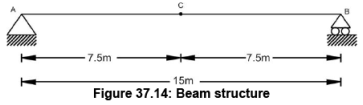Solution:
Tabulated values:

Place a unit load at different location between two supports and find the support reactions. Once the support reactions are computed, take a section at C and compute the moment. For example, we place the unit load at x=2.5 m from support A (Figure 37.15), then the support reaction at A will be 0.833 and support reaction B will be 0.167. Taking section at C and computation of moment at C can be given by

Σ Mc = 0 : - Mc + RB x 7.5 - = 0 ⇒ - Mc + 0.167 x 7.5 - = 0 ⇒ Mc = 1.25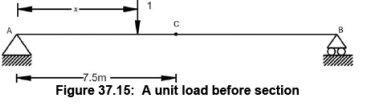Similarly, compute the moment Mc for difference unit load position in the span. The values of Mc are tabulated as follows.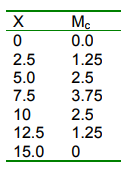Graphical representation of influence line for Mc is shown in Figure 37.16.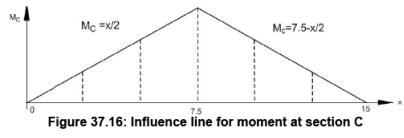Influence Line Equations:
There will be two influence line equations for the section before point C and after point C.

When the unit load is placed before point C then the moment equation for given Figure 37.17 can be given by

Σ Mc = 0 : Mc + 1(7.5 –x) – (1-x/15)x7.5 = 0 ⇒ Mc = x/2, where 0 ≤ x ≤ 7.5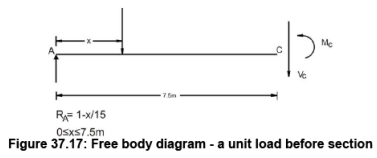When the unit load is placed after point C then the moment equation for given Figure 37.18 can be given by

Σ Mc = 0 : Mc – (1-x/15) x 7.5 = 0 ⇒ Mc = 7.5 - x/2, where 7.5 < x ≤ 15.0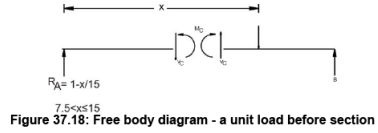The equations are plotted in Figure 37.16.

Example 5:
Construct the influence line for the moment at point C of the beam shown in Figure 37.19.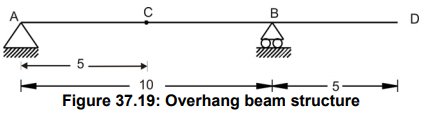Solution:
Tabulated values:

Place a unit load at different location between two supports and find the support reactions. Once the support reactions are computed, take a section at C and compute the moment. For example as shown in Figure 37.20, we place a unit load at 2.5 m from support A, then the support reaction at A will be 0.75 and support reaction B will be 0.25.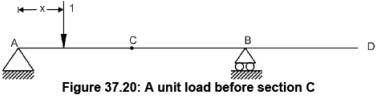Taking section at C and computation of moment at C can be given by

Σ Mc = 0 : - Mc + RB x 5.0 - = 0 ⇒ - Mc + 0.25 x 5.0 = 0 ⇒ Mc = 1.25

Similarly, compute the moment Mc for difference unit load position in the span. The values of Mc are tabulated as follows.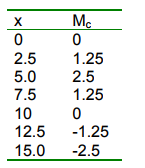Graphical representation of influence line for Mc is shown in Figure 37.21.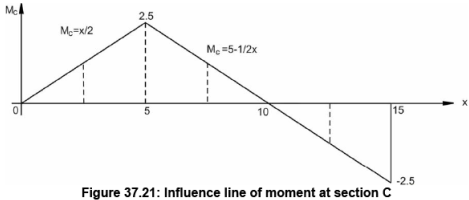Influence Line Equations:

There will be two influence line equations for the section before point C and after point C.

When a unit load is placed before point C then the moment equation for given Figure 37.22 can be given by

Σ Mc = 0 : Mc + 1(5.0 –x) – (1-x/10)x5.0 = 0 ⇒ Mc = x/2, where 0 ≤ x ≤ 5.0When a unit load is placed after point C then the moment equation for given Figure 37.23 can be given by

Σ Mc = 0 : Mc – (1-x/10) x 5.0 = 0 ⇒ Mc = 5 - x/2, where 5 < x ≤ 15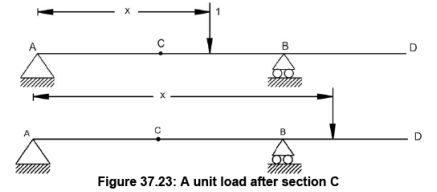The equations are plotted in Figure 37.21.

Influence line for beam having point load and uniformly distributed load acting at the same time

Generally in beams/girders are main load carrying components in structural systems. Hence it is necessary to construct the influence line for the reaction, shear or moment at any specified point in beam to check for criticality. Let us assume that there are two kinds of load acting on the beam. They are concentrated load and uniformly distributed load (UDL).

As shown in the Figure 37.24, let us say, point load P is moving on beam from A to B. Looking at the position, we need to find out what will be the influence line for reaction B for this load. Hence, to generalize our approach, like earlier examples, let us assume that unit load is moving from A to B and influence line for reaction A can be plotted as shown in Figure 37.25. Now we want to know, if load P is at the center of span then what will be the value of reaction A? From Figure 37.24, we can find that for the load position of P, influence line of unit load gives value of 0.5. Hence, reaction A will be 0.5xP. Similarly, for various load positions and load value, reactions A can be computed.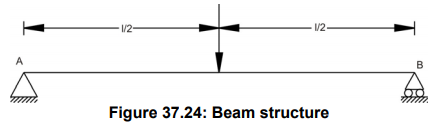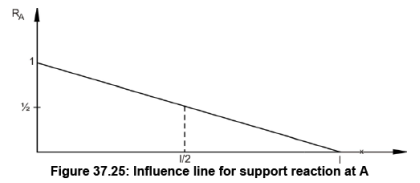Beam is loaded with uniformly distributed load (UDL) and our objective is to find influence line for reaction A so that we can generalize the approach. For UDL of w on span, considering for segment of dx (Figure 37.26), the concentrated load dP can be given by w.dx acting at x. Let us assume that beam’s influence line ordinate for some function (reaction, shear, moment) is y as shown in Figure 37.27. In that case, the value of function is given by (dP)(y) = (w.dx).y. For computation of the effect of all these concentrated loads, we have to integrate over the entire length of the beam. Hence, we can say that it will be ∫ w.y.dx = w ∫ y.dx. The term ∫ y.dx is equivalent to area under the influence line.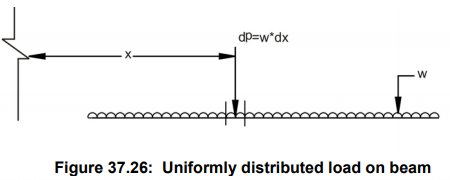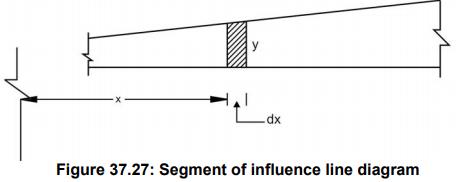For a given example of UDL on beam as shown in Figure 37.28, the influence line (Figure 37.29) for reaction A can be given by area covered by the influence line for unit load into UDL value. i.e. [0.5x (1)xl] w = 0.5 w.l.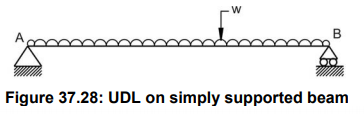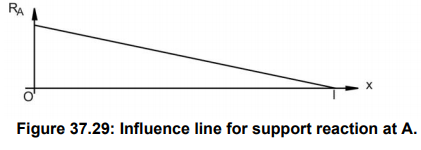Numerical Example

Find the maximum positive live shear at point C when the beam (Figure 37.30) is loaded with a concentrated moving load of 10 kN and UDL of 5 kN/m.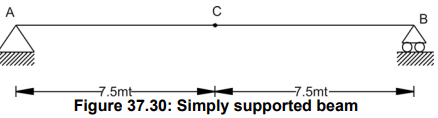Solution:
As discussed earlier for unit load moving on beam from A to B, the influence line for the shear at C can be given by following Figure 37.31.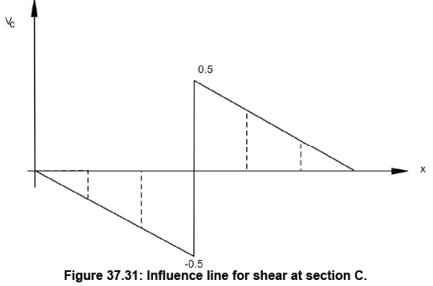Concentrated load: As shown in Figure 37.31, the maximum live shear force at C will be when the concentrated load 10 kN is located just before C or just after C. Our aim is to find positive live shear and hence, we will put 10 kN just after C. In that case,

Vc = 0.5 x 10 = 5 kN.

UDL: As shown in Figure 37.31, the maximum positive live shear force at C will be when the UDL 5 kN/m is acting between x = 7.5 and x = 15.

Vc = [0.5 x (15 –7.5) (0.5)] x 5 = 9.375

Total maximum Shear at C:

(Vc) max = 5 + 9.375 = 14.375.

Finally the loading positions for maximum shear at C will be as shown in Figure 37.32. For this beam one can easily compute shear at C using statics.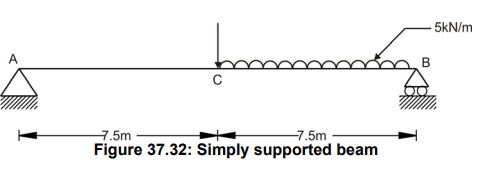Offer running on EduRev: Apply code STAYHOME200 to get INR 200 off on our premium plan EduRev Infinity!

## Structural Analysis

30 videos|122 docs|28 tests

,

,

,

,

,

,

,

,

,

,

,

,

,

,

,

,

,

,

,

,

,

;